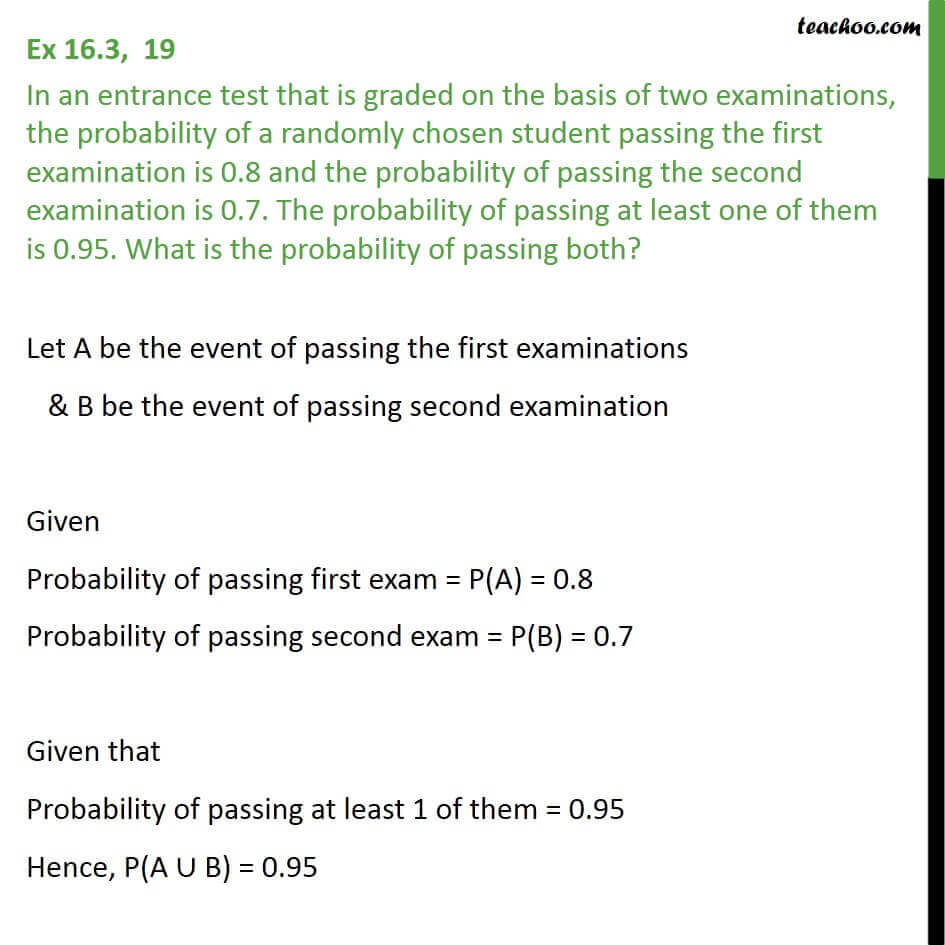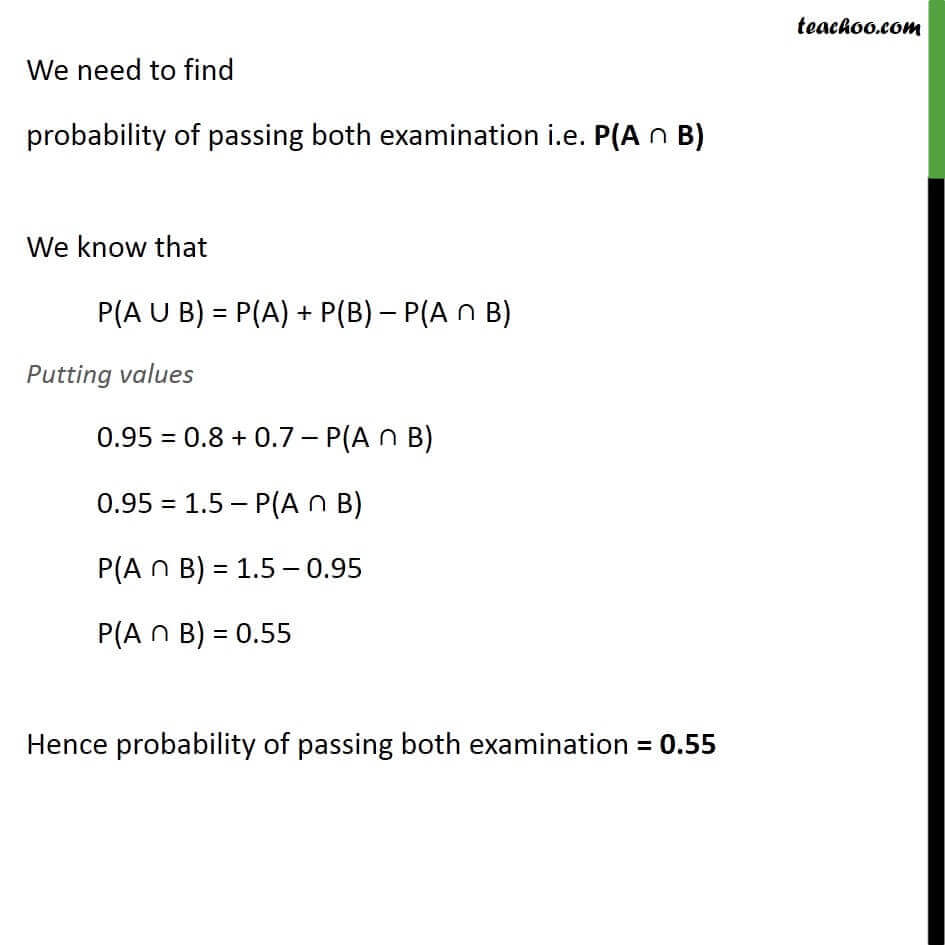Ex 14.2

Chapter 14 Class 11 Probability
Serial order wiseLearn in your speed, with individual attention - Teachoo Maths 1-on-1 Class

### Transcript

Ex 14.2, 19 In an entrance test that is graded on the basis of two examinations, the probability of a randomly chosen student passing the first examination is 0.8 and the probability of passing the second examination is 0.7. The probability of passing at least one of them is 0.95. What is the probability of passing both? Let A be the event of passing the first examinations & B be the event of passing second examination Given Probability of passing first exam = P(A) = 0.8 Probability of passing second exam = P(B) = 0.7 Given that Probability of passing at least 1 of them = 0.95 Hence, P(A ∪ B) = 0.95 We need to find probability of passing both examination i.e. P(A ∩ B) We know that P(A ∪ B) = P(A) + P(B) – P(A ∩ B) Putting values 0.95 = 0.8 + 0.7 – P(A ∩ B) 0.95 = 0.15 – P(A ∩ B) P(A ∩ B) = 0.55 Hence probability of passing both examination = 0.55